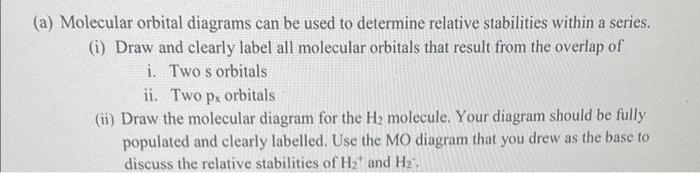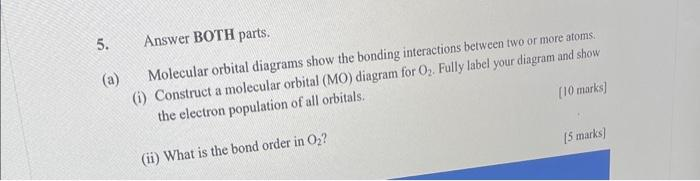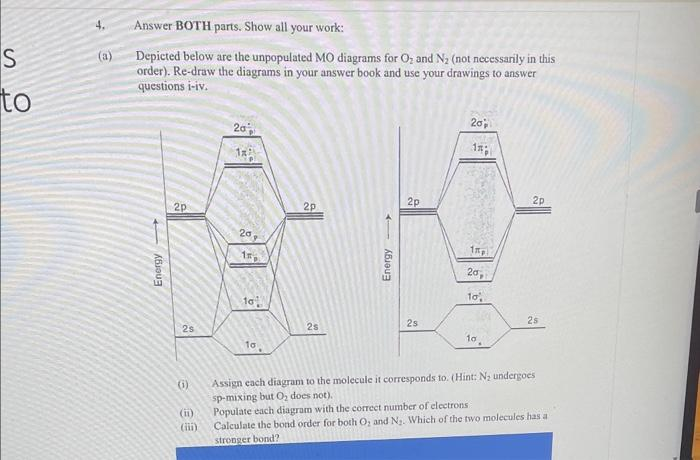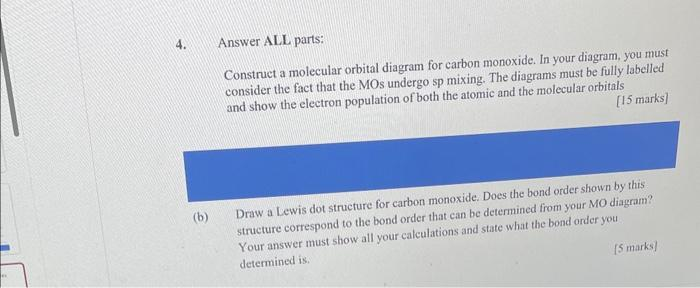Home / Expert Answers / Chemistry / a-molecular-orbital-diagrams-can-be-used-to-determine-relative-stabilities-within-a-series-i-pa450

# (Solved): (a) Molecular orbital diagrams can be used to determine relative stabilities within a series. (i) ...(a) Molecular orbital diagrams can be used to determine relative stabilities within a series. (i) Draw and clearly label all molecular orbitals that result from the overlap of i. Two s orbitals ii. Two px orbitals (ii) Draw the molecular diagram for the $$\mathrm{H}_{2}$$ molecule. Your diagram should be fully populated and clearly labelled. Use the MO diagram that you drew as the base to discuss the relative stabilities of $$\mathrm{H}_{2}^{+}$$and $$\mathrm{H}_{2}$$. 5. Answer BOTH parts. (a) Molecular orbital diagrams show the bonding interactions between two or more atoms. (i) Construct a molecular orbital (MO) diagram for $$\mathrm{O}_{2}$$. Fully label your diagram and show the electron population of all orbitals. [10 marks] (ii) What is the bond order in $$\mathrm{O}_{2}$$ ? [5 marks] Depicted below are the unpopulated MO diagrams for $$\mathrm{O}_{2}$$ and $$\mathrm{N}_{2}$$ (not necessarily in this order). Re-draw the diagrams in your answer book and use your drawings to answer questions i-iv. (i) Assign each diagram to the molecule it corresponds to. (Hint: $$\mathrm{N}_{2}$$ undergoes sp-mixing but $$\mathrm{O}_{2}$$ does not). (ii) Populate each diagram with the correct number of electrons (iii) Calculate the bond order for both $$\mathrm{O}_{2}$$ and $$\mathrm{N}_{2}$$. Which of the two molecules has a stronger bond? 4. Answer ALL parts: Construct a molecular orbital diagram for carbon monoxide. In your diagram. you must consider the fact that the MOs undergo sp mixing. The diagrams must be fully labelled and show the electron population of both the atomic and the molecular orbitals [15 marks] (b) Draw a Lewis dot structure for carbon monoxide. Does the bond order shown by this structure correspond to the bond order that can be determined from your MO diagram? Your answer must show all your calculations and state what the bond order you determined is. [5 marks]

We have an Answer from Expert